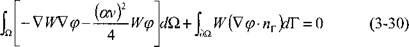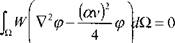## Finite Element Solutions with Prescribed Temperature

Of the three strong candidate numerical methods; finite difference, boundary element and finite element analysis, the finite element method have been chosen for its capability for nonlinear analysis and dealing with complex geometry. In addition, it is most compatible with modem CAD/CAM software systems. For thermal analysis alone, a strong argument can be made in favour of finite difference methods. However, for thermoplastic analysis the argument is stronger for finite element analysis. The boundary element method is not well developed for nonlinear analysis. Briefly, these considerations led to the choice of FEA as the most
effective numerical method for developing a complete analysis capability for computer modeling or simulation of welds.

The finite element method FEM usually imposes a piece-wise polynomial approximation of the temperature field within each element:

nodes

T(x, y,z, t)= ХхіУ^Уііґ) (3-25)

/=1

where Ni are basis functions dependent only on the type of element and its size and shape. Physically 7) (t) is the value of the temperature at node і at time t.

 dN dN; AV, ' ’ - V ' ’ І
 (3-26)

ЭГ ЭГ dr'

Эх ’ dy ’ dZ

Эх ' Э^ ' dz where ^ /V, (x, y, z)T: (?) is abbreviated to TV, 7).

The next question is how to evaluate Г,? Galerkin’s FEM is among the most convenient and general of the methods available for this purpose. If eq. (3-25) is substituted into eq. (3-2), a residual or error term must be added. If this was not true eq. (3-25) would be the exact solution. Indeed when eq. (3-25) is the exact solution, the error in the FEM solution is zero.

Galerkin’s FEM method requires:

£ £ Ni dQ= 0 (3-27)

Mathematically s is the residual from eq. (3-2); TV, in eq. (3-27) is a test function and the N{ terms in eq. (3-25) are the trial functions. Since there are і nodes, eq. (3-27) creates a set of і ordinary differential equations which are integrated to form a set of algebraic equations for each time step:

[К] [T] = [R] (3-28)

This set of equations is solved for the nodal temperatures T, at the end of the time step. Usually some form of Newton-Raphson method together with a Gaussian elimination and back substitution would be employed.

Applying Galerkin’s method to the equation (3-7), when Q is zero gives:

(3-29)

 for all W in the space of test functions. For infinite domains the test functions must be specified carefully but for a finite domain the usual Galerkin test functions can be used. Apply Green’s identity to equation (3-29):Applying the boundary condition in equation (3-22) to the side walls,V У(3-31)

If the boundary condition in equation (3-24) is applied on the downstream end,

 (3-32)

lw(S/<p-nTir = l^W<pnsdr.

2 2 £

 When the end faces are perpendicular to n^ = - 1 at the rear end. Putting equations (3-31) and (3-33) into (3-30):for all test functions W.

Комментарии закрыты.